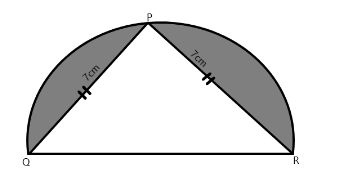The ΔPQR is inscribed in a semicircle. PQ = PR = 7cm . Find the area of the shaded region.

# The $\Delta$PQR is inscribed in a semicircle. PQ = PR = 7cm . Find the area of the shaded region.1. A
14
2. B
26
3. C
50
4. D
20

Fill Out the Form for Expert Academic Guidance!l

+91

Live ClassesBooksTest SeriesSelf Learning

Verify OTP Code (required)

### Solution:

The measure of the angle prescribed by the diameter of the semicircle on its circumference is 90°. So
∠QPR = 90°.
Therefore, $\Delta$PQR is a right-angled triangle.
Area of a right-angled triangle is,To find the area of the semicircle, we have to find out its radius first.
As $\Delta$PQR  is a right-angled triangle, so by applying Pythagoras theorem, we getcmis the diameter of the semicircle, we know that radius is half of the diameter of the circle, socm.
The area of the semicircle isThe area of the shaded region isHence the area of the shaded region is 14 $c{m}^{2}$.
Hence, Option (1) is the correct answer.

## Related content

 Area of Square Area of Isosceles Triangle Pythagoras Theorem Triangle Formula Perimeter of Triangle Formula Area Formulae Volume of Cone Formula Matrices and Determinants_mathematics Critical Points Solved Examples Type of relations_mathematics+91

Live ClassesBooksTest SeriesSelf Learning

Verify OTP Code (required)